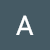# Create Machine Learning Model

Now, let first understand What is Linear Regression?

1. Does a set of predictor variables do a good job in predicting an outcome (dependent) variable?
2. Which variables in particular are significant predictors of the outcome variable, and in what way do they–indicated by the magnitude and sign of the beta estimates–impact the outcome variable?

The simplest form of the regression equation with one dependent and one independent variable is Linear Regression i.e. defined by the formula y = b+w*x

Now, we also have to Install some of the depended libraries inside container that are give below

# The Wrap-Up

--

--## Abhayagarwal

I am tech enthusiast fascinated towards technology and its various disciplines including Big Data, Hadoop, Web Development, Competative Programming,ML,etc.